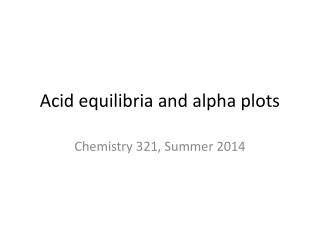Download PresentationAcid equilibria and alpha plots# Acid equilibria and alpha plots - PowerPoint PPT Presentation

Download Presentation##### Acid equilibria and alpha plots

Download Policy: Content on the Website is provided to you AS IS for your information and personal use and may not be sold / licensed / shared on other websites without getting consent from its author. While downloading, if for some reason you are not able to download a presentation, the publisher may have deleted the file from their server.

- - - - - - - - - - - - - - - - - - - - - - - - - - - E N D - - - - - - - - - - - - - - - - - - - - - - - - - - -
##### Presentation Transcript

1. Acid equilibria and alpha plots Chemistry 321, Summer 2014

2. In this lecture • Alpha plots show dynamic changes in species concentration during a titration • For weak acids, alpha plots mirror the behavior seen on titration plots and yield further information

3. Formal concentration Given: Let CHA = formal concentration of the acid = [HA] + [A–] = sum of all forms of the acid at equilibrium CHA = [HA]0 if only the weak acid is added = [A–]0if only the conjugate base is added = [HA]0 + [A–]0 if both are added

4. Alpha is the mole fraction of a species relative to the solution’s formal concentration So, symbolically, For instance, We can rewrite this like: [HA] = αHA CHAor [A–] = = αA– CHA The constraint is that Σ αi = 1 For a monoprotic acid, αHA + αA– = 1

5. The equation of the alpha plot Start with the definitions of CHA and αHA:

6. The equation of the alpha plot Start with the definitions of CHA and αHA: Invert the expression:

7. The equation of the alpha plot Start with the definitions of CHA and αHA: Invert the expression: Recalling the equilibrium expression: so

8. The equation of the alpha plot (continued) Re-invert the equation: Similarly, we can derive an expression for αA–: Note that both expressions for alpha depend on [H+] (and, by extension, pH) only! So this can be plotted.

9. The alpha plot pKa Where the two curves cross (each α = 0.5), the x-coordinate is the pKaof the weak acid.

10. Limiting behavior on the graph For acetic acid, Ka = 1.8 × 10–5. Consider an acetic acid solution at pH 8.74 (meaning [H+] = 1.8 × 10–9). The point is that even at a really high pH, there is still someundissociated acid left (i.e., not zero). When you are dealing with a polyproticacid or base, then there are pHs at which some species have zero concentration…this is also important when dealing with ligands.

11. Polyprotic acid alpha plots Polyprotic acid dissociation occurs in a stepwise manner; that is, the different H+ ions on each molecule dissociate at different pHs, rather than all at once. Note the definitions of the equilibrium constants Ka1 and Ka2 N.B.: pKa1 = 6.37, and pKa2 = 10.25

12. Setting up the alpha plot equations for a diprotic acid Let CH2A = [H2A] + [HA–] + [A2–] ( = formal concentration of H2A) Expanding the previous definition of αi: [H2A] = αH2A CH2A and [HA–] = αHA– CH2A and [A2–] = αA2- CH2A Note that αH2A + αHA– + αA2- = 1

13. Setting up the alpha plot equations for a diprotic acid Let CH2A = [H2A] + [HA–] + [A2–] ( = formal concentration of H2A) Expanding the previous definition of αi: [H2A] = αH2A CH2A and [HA–] = αHA– CH2A and [A2–] = αA2- CH2A Note that αH2A + αHA– + αA2- = 1

14. Setting up the alpha plot equations for a diprotic acid Inverting the equation yields:

15. Setting up the alpha plot equations for a diprotic acid Inverting the equation yields: Recall the definitions of Ka1 and Ka2:

16. Setting up the alpha plot equations for a diprotic acid Inverting the equation yields: Recall the definitions of Ka1 and Ka2: multiply to get:

17. Setting up the alpha plot equations for a diprotic acid Substitute into the original expression:

18. Setting up the alpha plot equations for a diprotic acid Substitute into the original expression: Put it all over a common denominator: Limiting behavior: at low pH (acidic), the [H+]2 term dominates the other terms, so αH2A ≈ 1; at high pH (alkaline), [H+]2 0, so αH2A ≈ 0.

19. Setting up the alpha plot equations for a diprotic acid The other αi expressions (αHA– and αA2–) are derived similarly.

20. The alpha plot for carbonic acid What are the pKas for carbonic acid?

21. The alpha plot for carbonic acid At pH 4, αCO3 2- ≈ 0, according to the graph

22. The alpha plot for carbonic acid not zero, but basically negligible! At pH 4, the only two species that matter are HCO3–and H2CO3

23. The titration curve for carbonic acid reflects the stepwise dissociation behavior

24. Challenge problem Consider the stepwise dissociation of phosphoric acid:

25. Challenge problem (continued) • Derive the equations to calculate αi for all phosphate-containing species. • Draw the alpha plot for all phosphate-containing species; make sure the axes are labeled and the pKas are sensible. • Calculate the mole fraction (αi) for all phosphate-containing species at blood pH (7.40). • Assume all phosphate-containing species have a soluble sodium salt (i.e., if you need PO43–, you will use Na3PO4). Which two salts will you use to create pH 7.40 phosphate buffer?